Home > A2C > Chapter 9 > Lesson 9.2.2 > Problem9-96

9-96.
1.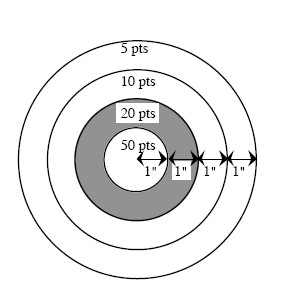In one of the games at the county fair, people pay to shoot a paint pistol at the target shown below right. The center has a radius of one inch. Each concentric circle has a radius one inch larger than the preceding circle. Assuming the paint pellet hits the target randomly, what is the probability that it hits: Homework Help ✎

1. The 50-point ring?

2. The 20-point ring?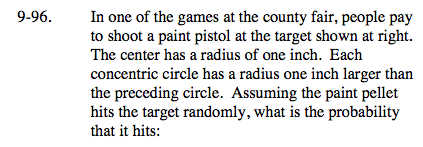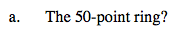Area of a circle = πr2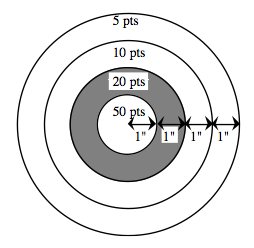$P(50pt)=\frac{\text{area of success}}{\text{total area}}=\frac{\pi(1)^{2}}{\pi(4)^{2}}$

$\frac{1}{16}$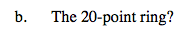$P(20pt)=\frac{\text{area of success}}{\text{total area}}=\frac{\pi(2)^{2}-\pi(1)^{2}}{\pi(4)^{2}}$